# “中一千万”与“挣一千万”到底哪个更容易

· 阅读需 19 分钟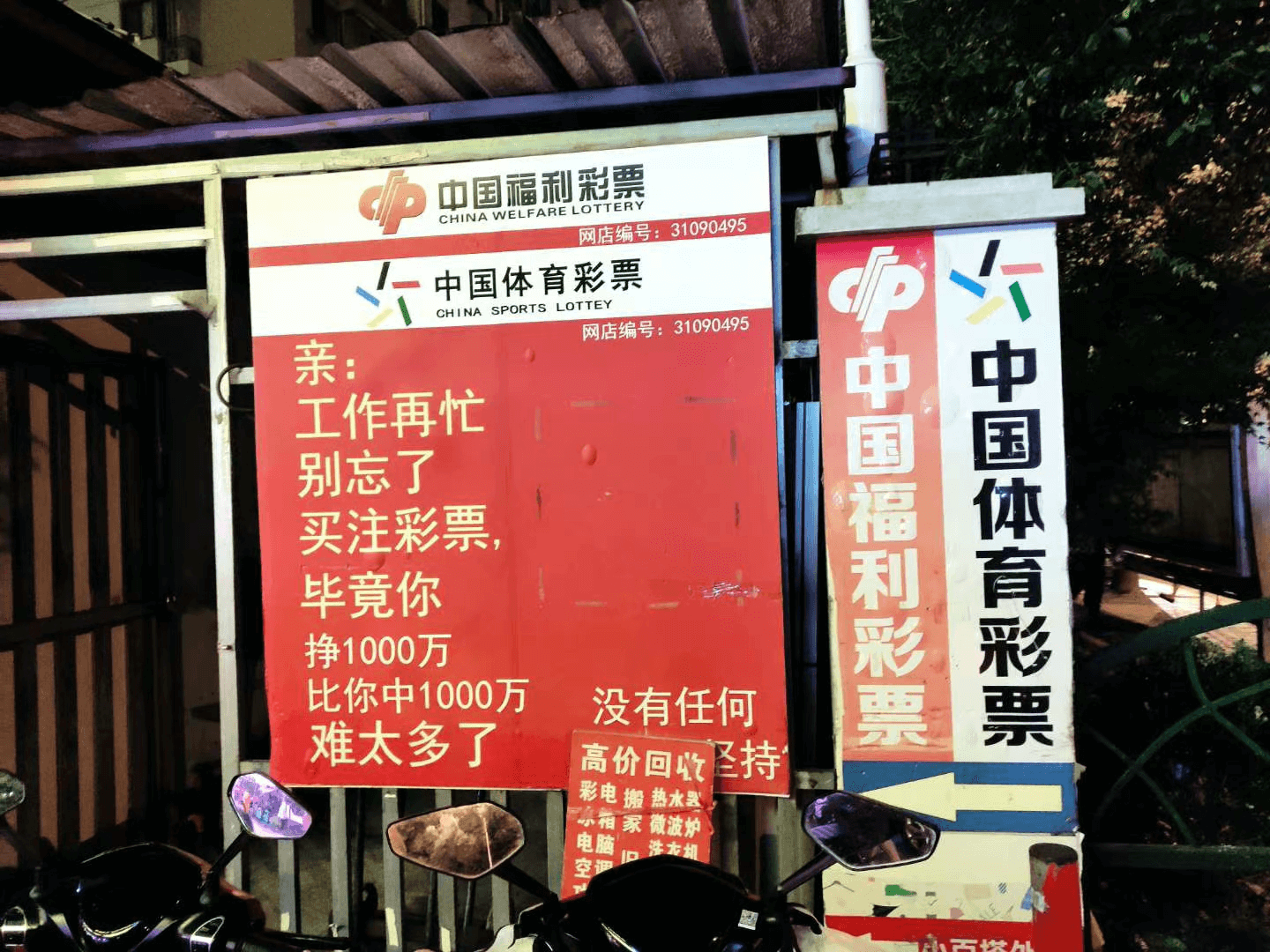## 大乐透模型搭建与分析​

### 大乐透的中奖概率分析​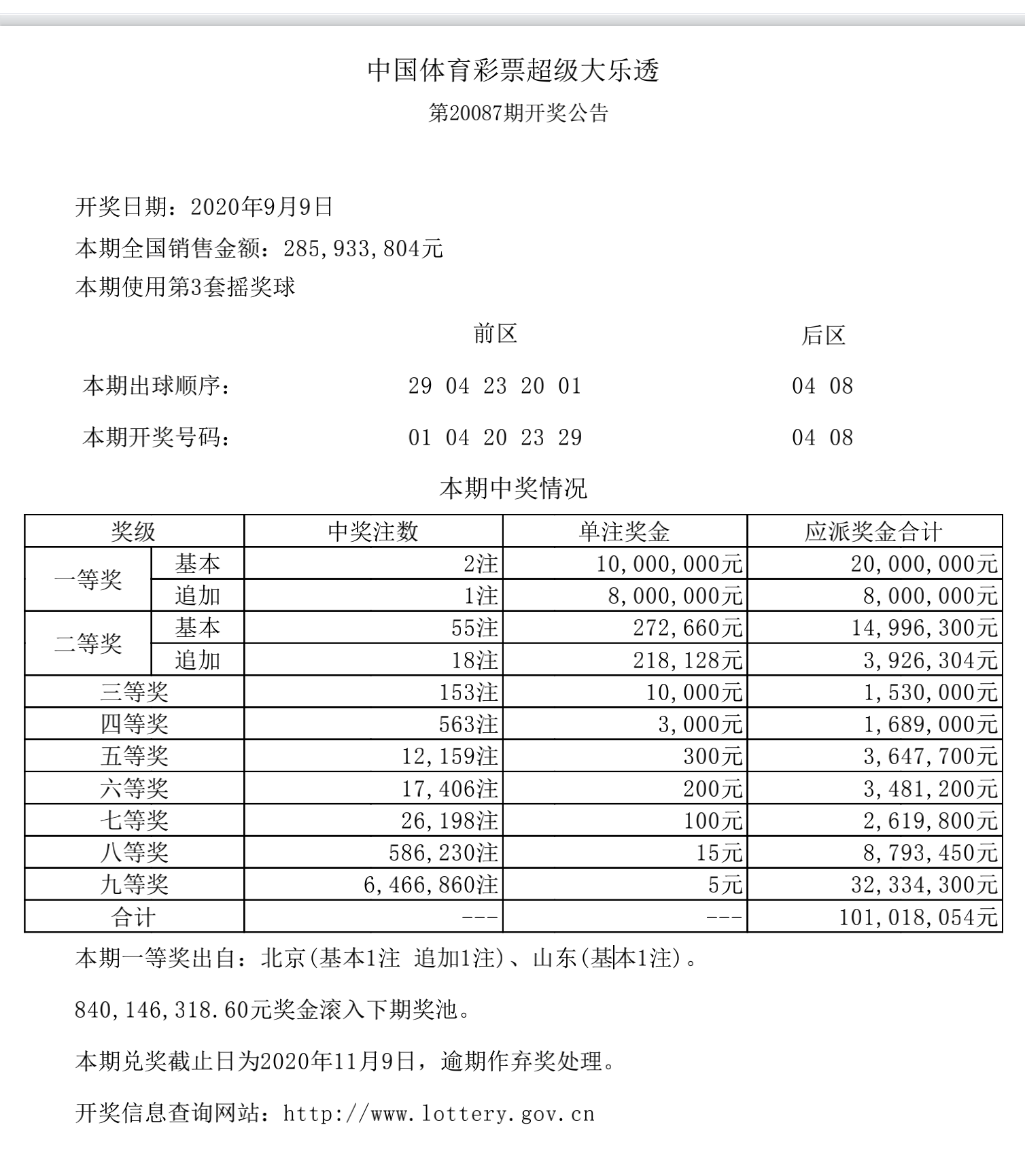### 大乐透的投资模型搭建​

1. 若中奖，我们的本金变成 $a_0*(1-k)+q$。
2. 若没有中奖，我们的本金变成 $a_0*(1-k)$。

1. 若中奖，我们的本金变成 $10*(1-k)+1000$。
2. 若没有中奖，我们的本金变成 $10*(1-k)$。

1. 你会购买至少一注大乐透吗？用大概率会浪费的 N 元，去博取 1000w？
2. 你会长时间的购买 N 注大乐透吗？为什么？

### 大乐透的投资模型分析（一）：按比率投资模型​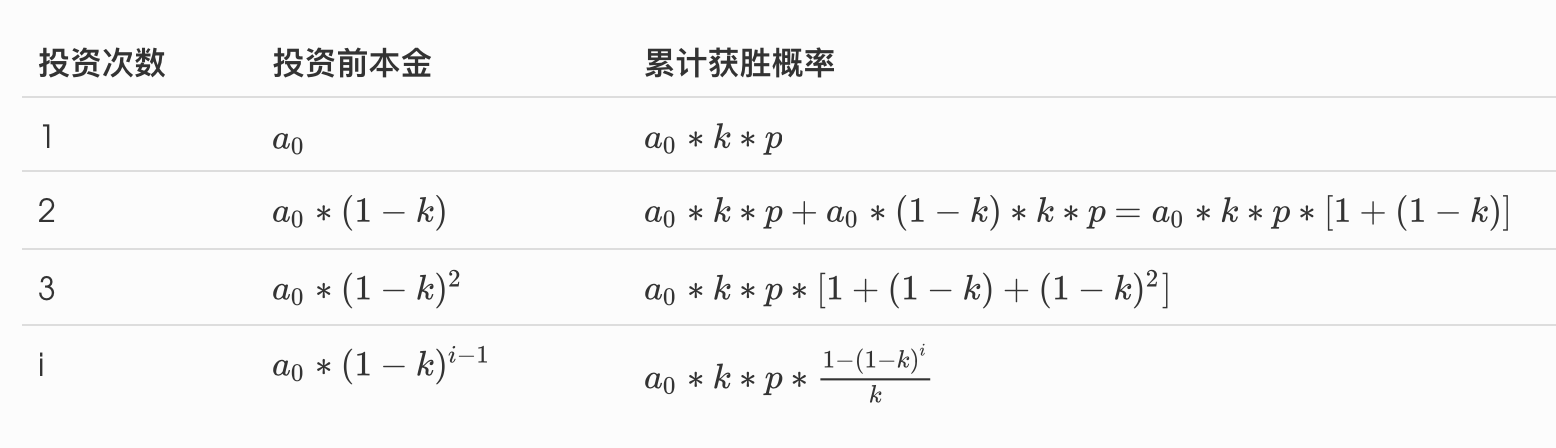1$a_0$$a_0kp 2a_0*(1-k)$$a_0kp + a_0(1-k)kp$ $=a_0kp[1+(1-k)]$
3$a_0*(1-k)^2$$a_0kp*[1+(1-k)+(1-k)^2] ia_0*(1-k)^{i-1}$$a_0kp*\frac{1-(1-k)^i}{k}$

## 另一种看起来简单点的赌注模型​

### 基于目标收益的确定性投资​

$0.5 (100-x+3x) + 0.5(100-x) = 100 * (1+0.1)$

import randomimport numpy as npimport pandas as pdimport matplotlib.pyplot as pltimport seaborn as snsplt.rcParams['font.sans-serif'] = ['SimHei', 'STHeiti'] #显示中文标签plt.rcParams['axes.unicode_minus'] = FalseA = 100     # 本金（单位：万）P = 0.5     # 中奖概率R = 3       # 中奖赔率T = 11      # 参与者个数LU = 1      # 10的指数上限LD = 0      # 10的指数下限N = 10      # 赌注次数def simulate_by_ratio(k):    cur_asset = A    assets = [cur_asset]    for day in range(N):        pay = cur_asset * k        cur_asset -= pay        if random.random() < P:            cur_asset += pay * R        assets.append(cur_asset)    return assetsarr = [simulate_by_ratio(k) for k in np.linspace(LD, LU, T)]data = pd.DataFrame(arr).Tdata.columns = ['{: 3d}%'.format(int(100*k)) for k in np.linspace(LD, LU, T)]title = f'本金:{A}, 中奖概率: {P}, 中奖赔率: {R}, 赌注次数: {N}, 参与者：{T}'ax = sns.relplot(data=data, kind='line', dashes=False).set(title=title)ax._legend.set_title('投资比率')plt.show()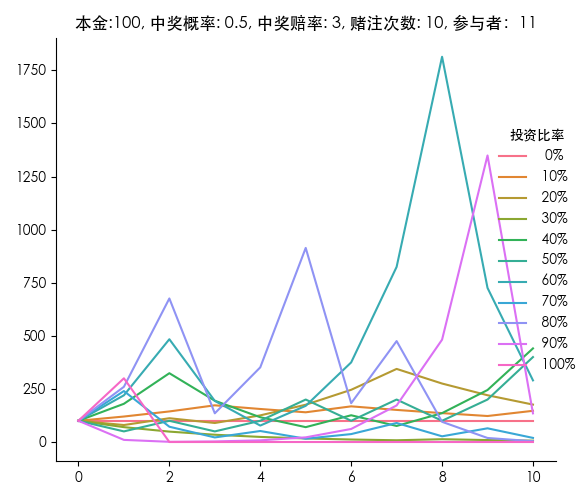### 基于长期最大期望收益的确定性投资​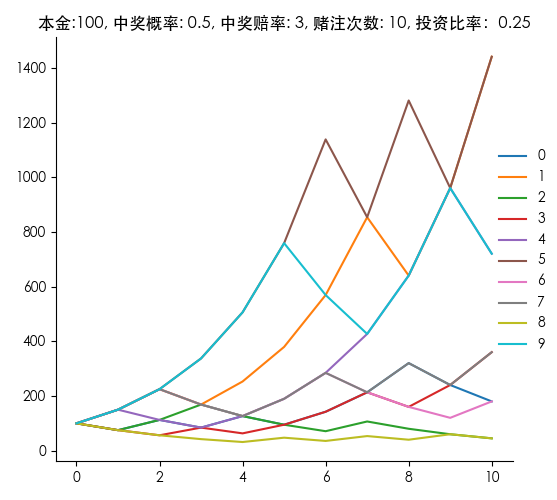$A_N = A_0 (1+kq)^{Np} (1+kr)^{N(1-p)}$，对数后得

$ln(A_N) = ln(A_0) + N (pln(1+kq)+(1-p)*ln(1+kr))$，即求

$max\ f(k) = p ln(1+kq) + (1-p) ln(1+kr)$，对其求导，则有

$max f'(k) = \frac{pq}{1+kq} + \frac{(1-p)r}{1+kr}$，显然直接令其等于 0 即可得到函数拐点，即

$（*）\frac{pq}{1+kq} + \frac{(1-p)r}{1+kr}=0$，则有

$（）k=\frac{pq+(1-p)r}{-qr}$，其中分子就等于胜率乘以胜赔率加上输率乘以输赔率，分母则为负的胜率乘以赔率**。

$（***）k=\frac{p(q+1)-1}{q}$

## 使用凯利公式重买大乐透​